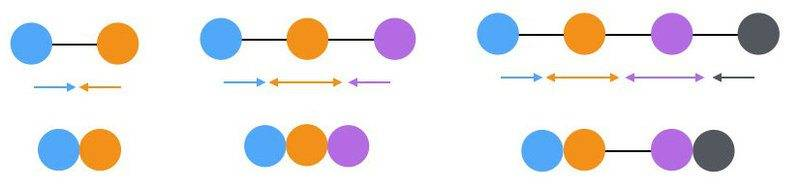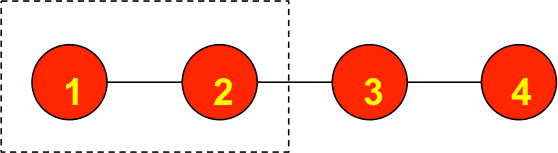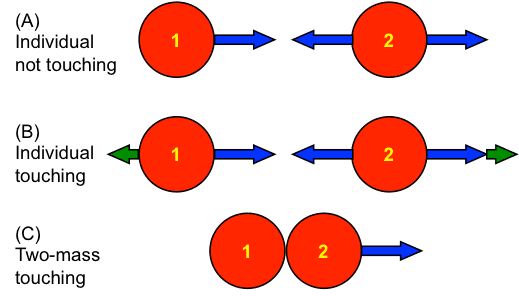# Pulling problem

Member has been warned not to delete the template.

## Homework Statement

Imagine exactly same two bodies (same mass, same surface/friction etc) are attached with a string/spring and they suddenly start pulling towards each other with equal force. I can imagine they will move towards the center. If there is a chain (with defined number; not infinite) of such bodies attached to each other and each body starts pulling towards each other then would whole system shrink towards the center or just the bodies at the terminal ends because rest of the bodies are experiencing equal and opposite forces. I am stuck.

2. Homework Equations

## The Attempt at a Solution

Posted free body diagram in later reply {edited myself}

Last edited:

scottdave
Homework Helper
Gold Member
Are these in a straight line? Consider one of the masses at either end. What forces are acting on these? Which direction will they move? Could you make a free body diagram for each one to get the net force on each one? Start with 2. What about 3, 4. Is there a pattern?

•sagarkumar
Are these in a straight line? Consider one of the masses at either end. What forces are acting on these? Which direction will they move? Could you make a free body diagram for each one to get the net force on each one? Start with 2. What about 3, 4. Is there a pattern?

Thanks, I have considered most of them already and showing here in image . Arrows show forces direction. Still I think only terminal bodies will move. Not sure though if am missing any other aspect.#### Attachments

kuruman
Homework Helper
Gold Member
2021 Award
Are there external forces acting on any component of the system of masses?
Can the center of mass of the system accelerate if no external forces act on any of the masses?

kuruman
Homework Helper
Gold Member
2021 Award
Thanks, I have considered most of them already and showing here in image . Arrows show forces direction. Still I think only terminal bodies will move. Not sure though if am missing any other aspect.

View attachment 227756
Look at the figure on the lower right. You have four masses that can be considered as two masses ##2m## pulling on each other. Will they stay where they are or move together?

•scottdave and sagarkumar
Look at the figure on the lower right. You have four masses that can be considered as two masses ##2m## pulling on each other. Will they stay where they are or move together?

Yes they should shrink towards each other 'if' forces are acting constantly. 'But' the way I was thinking that forces are applied at the same and for one instant only then goes off.
Again, yes they will move towards each other but now as the mass is increased the displacement would be less (halved in this case). So if constant force keeps on acting then can I say that the system starts shrinking from outside towards the inside?

kuruman
Homework Helper
Gold Member
2021 Award
So if constant force keeps on acting then can I say that the system starts shrinking from outside towards the inside?
The answer to this question is obvious if you first answer my question is post #4, "Can the center of mass of the system accelerate if no external forces act on any of the masses?"

The answer to this question is obvious if you first answer my question is post #4, "Can the center of mass of the system accelerate if no external forces act on any of the masses?"

Nothing is obvious :) To answer your question: No it will not (to correct it 'if no net external forces acting'). But how you are trying to relate this to what I said about shrinking process starts from the outside; I mean it does not give any visual explanation.

kuruman
Homework Helper
Gold Member
2021 Award
Say you start with a linear chain of 4 equal masses and equal constant forces between adjacent pairs of masses. Mass 1 experiences a net force to the right. So the distance between 1 and 2 will decrease. However, you can consider masses 1 and 2 together as a system acted upon by an external force to the right due to the system consisting of masses 3 and 4 (see figure below). So the distance between 2 and 3 will also decrease. By symmetry, the distance between 3 and 4 will decrease just like the distance between 1 and 2. All separations between masses decrease at the same time. A visual demonstration of this would be to zoom out your screen while watching the figure below. Concentrate on the separations between circles and pretend that the diameter of the circles stays the same.#### Attachments

•Delta2
sagarkumar: As you might see, kuruman is applying the general principle that internal forces cannot affect the position of the center of mass of a body (or of a system of bodies). This can be seen as a consequence of the first law of motion or of the law of conservation of momentum. That gives a direct path to your initial question:
would whole system shrink towards the center or just the bodies at the terminal ends because rest of the bodies are experiencing equal and opposite forces
It is not clear to me if you see what that analysis tells you about the how the masses end up. What you wrote in post #6 suggests you think there will be a symmetric collapse toward the center of mass.

kuruman: I think your analysis is an elegant example of finding parallels between the behavior of particles and groups of particles. I will be stealing this for my high school physics course.

As I consider all this, it seems to me the nature of the forces acting on the masses are presented in three ways:
1) Springs act (in the original post), so the magnitude of the force between each ball and its neighbor decreases proportionally to the separation of the balls.
2) The "forces are applied at the same and for one instant only then goes off" (post #6). That can be achieved through small explosions on the left and right of every mass except the terminal masses, which would have explosions only on the terminal sides. There is a very brief, simultaneous initial acceleration for the terminal balls and the others initially remain at rest.
3) A "constant force keeps on acting" (also post #6), so it is like having opposing rockets attached each side of each interior that fire constantly. There is a continuous acceleration of the terminal balls while the others initially remain at rest.

The end result is the same in all three cases, but sagarkumar refined the initial question to ask about how the collapse to the center of mass proceeds.

Which case are you using in your answer to the refined question? You conclude that the collapse is simultaneous throughout the system, whereas the second and third cases I presented (and the first if you take into account the time needed for the the decrease in tensions to move radially inward) make me think only the terminal balls move initially.

Say you start with a linear chain of 4 equal masses and equal constant forces between adjacent pairs of masses. Mass 1 experiences a net force to the right. So the distance between 1 and 2 will decrease. However, you can consider masses 1 and 2 together as a system acted upon by an external force to the right due to the system consisting of masses 3 and 4 (see figure below). So the distance between 2 and 3 will also decrease. By symmetry, the distance between 3 and 4 will decrease just like the distance between 1 and 2. All separations between masses decrease at the same time. A visual demonstration of this would be to zoom out your screen while watching the figure below. Concentrate on the separations between circles and pretend that the diameter of the circles stays the same.

View attachment 227758
Hi Kuruman,
I think its getting more complicated for me to understand it. Lets break down the process in terms of differentials of time snapshots, and lets consider 1, 2, 3 and 4 as individual masses rather combining any of them (e.g 1+2 etc.). At the very initial time dt masses 2 and 3 has net zero force but 1 will move towards 2 with force of k.x12 where k is force constant and x12 is distance between 1 and 2 (and similarly 4 will move towards 3). Thus at this initial dt step the distance between 1 and 2 has been decreased slightly but distance between 2 and 3 does not. Now at next time step dt as distance between 1 and 2 is decreased the k.x12 is also less thus net force on 2 is imbalanced and also at this same time step k.x23 is stronger to pull 2 towards 3. Does it makes sense please correct me? If yes then would not it be correct to say that shrinking really starts from the terminal ends? though the time scale am talking about is infinite small.

haruspex
Homework Helper
Gold Member
However, you can consider masses 1 and 2 together as a system acted upon by an external force to the right due to the system consisting of masses 3 and 4 (see figure below). So the distance between 2 and 3 will also decrease.
Not so.
The mass centre of the 1+2 system will move to the right, but that will be entirely down to the fact that mass 1 is moving to the right. If all tensions are equal and constant then mass 2 will not move until mass 1 reaches it.
This is counterintuitive because real springs do not maintain constant tension. In practice, as soon as mass 1 has moved a little mass 2 will accelerate.

I woke up this morning thinking that one arm of the line is approximately the same physics as a falling slinky.

kuruman
Homework Helper
Gold Member
2021 Award
Not so.
The mass centre of the 1+2 system will move to the right, but that will be entirely down to the fact that mass 1 is moving to the right. If all tensions are equal and constant then mass 2 will not move until mass 1 reaches it.
This is counterintuitive because real springs do not maintain constant tension. In practice, as soon as mass 1 has moved a little mass 2 will accelerate.
I agree. When I posted #9 I mentally switched to springlike forces.
Consider the (unrealistic) model of a constant force between adjacent masses and the FBD of a system being a "standalone" mass having a non-touching neighbor on either side. Below I show
(A) two separate FBDs of each mass when they are not touching. The accelerations are ##a_1=\frac{F}{m},~a_2=0##.
(B) two separate FBDs of each mass when they are touching. There is an additional contact force ##N## (green arrows) acting on each mass.
(C) a single FBD of the two-mass system. The forces in it are the vector sum of the forces in the two (B) diagrams.
From (C) the common acceleration, also the acceleration of the cm, is ##a_{cm}=\frac{F}{2m}##. Then from either diagram in (B) the magnitude of the contact force is ##N=\frac{F}{2}##.
So it looks like, under this model, the chain collapses from the ends in because mass 2 does not accelerate until it is touched by 1.#### Attachments

•sagarkumar
So it looks like, under this model, the chain collapses from the ends in because mass 2 does not accelerate until it is touched by 1.
View attachment 227778

Hi All,
I think we do have different explanations but we agree, and as nicely summarised by kuruman above, that shrinking starts from the terminal ends. I had been trying to explain this to my friends but no one agreed. Thanks kuruman, scottdave, haruspex, fewmet and PF for getting involved in the discussion.

haruspex
Homework Helper
Gold Member
I woke up this morning thinking that one arm of the line is approximately the same physics as a falling slinky.
That would be true if the links between the particles behave like springs (of zero relaxed length) with the tension being proportional to the length. But the model stated in this thread is that the tensions are constant over time.

kuruman